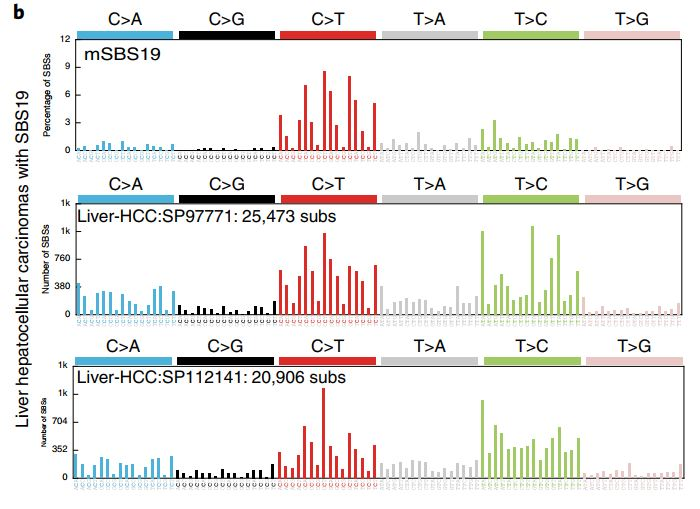# Mutation signature upstream and downstream base bar diagram drawing

#### Introduction

Show the mutation signal of a sample

#### Code explanation

##### Load the package, read in the
``````library(MutationalPatterns)

Its input data’nhdp’ part is as follows, each row is the corresponding upstream and downstream clips, and each column is the signal type

``````> str(nhdp)
num [1:96, 1:11] 0.01985 0.01188 0.00248 0.01138 0.01724 ...
- attr(*, "dimnames")=List of 2
..\$ : chr [1:96] "1" "2" "3" "4" ...
..\$ : chr [1:11] "SBS5" "SBS40" "SBS19" "SBS_N1" ...``````

The author originally used SigprofilerPlotting for drawing in Python, and later used the plot_96_profile function of the R package MutationalPatterns

``````#I used SigprofilerPlotting in python, however here I obtain the same results using MutationalPatterns
#for Figure 5b
#I want to show mSBS19 and some liver samples where I found with SBS19

signb=cbind(nhdp[,3],humandata[,c('Liver-HCC::SP97771','Liver-HCC::SP112141')])
colnames(signb)=c('mSB19','Liver-HCC:SP97771','Liver-HCC:SP112141')

fig5b<-plot_96_profile(signb,ymax=0.09)

ggsave(plot=fig5b, file='figure-5b.pdf', device=cairo_pdf, width=7, height=5)
main_layer <- theme_grey()+theme(axis.text.x = element_text(angle = 90, vjust = 0.5, hjust=1,size=7,color=1))``````mSBS19 and two spectra of human hepatocellular carcinomas where SBS19 was identified are shown.

#### Interpretation of results

Shows SBS19 signals identified in human cancer samples

#### References

Riva, L., Pandiri, A. R., Li, Y. R., Droop, A., Hewinson, J., Quail, M. A., … Adams, D. J. (2020). The mutational signature profile of known and suspected human carcinogens in mice. Nature Genetics. doi:10.1038/s41588-020-0692-4

#### Original code

https://github.com/team113sanger/mouse-mutatation-signatures